# Estimating Reservoir Porosity: Probabilistic Neural Network Estimation of Reservoir Porosity Using Probabilistic Neural Network Keywords: Porosity Seismic Attributes Probabilistic Neural Network (PNN) Highlights: Porosity is estimated from seismicattributes using Probabilistic Neural Networks. Impedance is calculated by using Probabilistic Neural Networks inversion. Multi-regression analysis is used to select input seismic attributes. Abstract Porosity is the most fundamental property of hydrocarbon reservoir. However, the porosity data that come from well log are only available at well points. Therefore, it is necessary to use other methods to estimate reservoir porosity. Interpolation is a simple and widely used method for porosity estimation. However, the accuracy of interpolation method is not satisfactory especially in the place where the numbers of wells are small. Seismic data contain abundant lithology information. There are inherent correlations between reservoir propertyand seismic data. Therefore, it ispossible to estimate reservoir porosity by using seismic data andattributes. Probabilistic Neural Network is a neoteric neuralnetwork modelbased on statistical theory.It is a powerful tool to extract mathematic relation between two data sets. For this case, it has been used to extract the mathematic relation between porosity and seismic attributes. In this study, firstly, a seismic impedance volume is calculated by seismic inversion. Secondly, several appropriate seismic attributes are extracted by using multi-regression analysis. Then, a Probabilistic Neural Network model is trained to obtain mathematic relation between porosity and seismic attributes. Finally, this trained Probabilistic Neural Network model is applied to calculate a porosity data volume. This methodology could be used to find advantageous areas at the early stage of exploration. And it is also helpful for the establishment of reservoir model at the stage of reservoir development. 1. Introduction In recent years, clear advances have been made in the study and application of intelligent systems. Intelligent system is a powerful tool to extract quantitative formulation between two data sets and has begun to be applied to the petroleum industry (Asoodeh and Bagheripour, 2014; Tahmasebi and Hezarkhani, 2012; Karimpouli et al., 2010; Chithra Chakra et al., 2013). There are inherent correlations between reservoir properties and seismic attributes (Iturrarán-Viveros and Parra, 2014; Yao and Journel, 2000). Therefore, it ispossible to estimate reservoir porosities by using seismic data and attributes. Previous studies have proved that it is feasible to estimate reservoir porosity by using statistical methods and intelligent systems (Na’imi et al., 2014; Iturrarán-Viveros, 2012; Leite and Vidal, 2011). Probabilistic NeuralNetwork (PNN) is a neoteric neural network model based on statistical theory. It is essentially a kind of parallel algorithm based on the minimum Bayesian risk criterion (Miguez, 2010). It is unlike traditional multilayer forward network that requires an error back propagation algorithm, but a completely forward calculation process. The training time is shorter and the accuracy is higher than traditional multilayer forward network. It is especially suitable for nonlinear multi attributes analysis. For this case, PNN has good performance on unseen data. In this study, the propounded methodology is applied to estimate the porosity of sandstone reservoir prosperously. 2. Probabilistic Neural Network PNN is a variant of Radial Basis Function networks and approximate Bayesian statistical methods, the combination of new input vectors with the existing data storage to fully classify the input data; a process that similar to human behavior (Parzen, 1962). Probabilistic Neural Network is an alternative type Neural Network (Specht, 1990). It is based on Parzen’s Probabilistic Density Function estimator. PNN is a four-layer feed-forward network, consisting of an input layer, a pattern layer, a summation layer and an output layer (Muniz et al., 2010). Probabilistic NeuralNetwork is actuallya mathematical interpolation method, but it has a structure of neural network. It has better interpolation function than multilayer feed forwardneural network. PNN’s requirement of training data sample is as same as Multilayer Feed Forward Neural Network. It includes a series of training sample sets, and each sample corresponds to the seismic sample in the analysis window of each well. Suppose that there is a data set of n samples, each sample consists of m seismic attributes and one reservoir parameter. Probabilistic Neural Network assumes that each output log value could be expressed as a linear combination of input logging data value (Hampson et al., 2001). The new sample after the attribute combination is expressed as: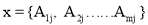(1) The new predicted logging values can be expressed as: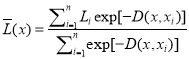(2) whereï¼š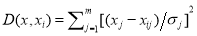(3) The unknown quantity D(x, xi) is the “distance” between input point and each training sample point. This distance is measured by seismic attributes in multidimensional space and it is expressed by the unknown quantity σj. Eq. (1)and Eq. (2) represent the application of Probabilistic Neural Network. The training process includes determining the optimal smoothing parameter set. The goal of the determination on these parameters is to make the validation error minimization. Defining the kth target point validation result as follows: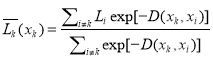(4) When the sample points are not in the training data, it is the kth target sample prediction value. Therefore, if the sample values are known, we can calculate the prediction error of sample points. Repeat this process for each training sample set, we can define the total prediction error of training data as: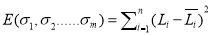ã€€ã€€ ã€€(5) The prediction error depends on the choice of parameter σj. This unknown quantity realizes the minimization through nonlinear conjugate gradient algorithm. Validation error, the average error of all excluded wells, is the measure of a possible prediction error in the process of seismic attributes transformation. The trained Probabilistic Neural Network has the characteristics of validation minimum error. The PNN does not require an iterative learning process, which can manage magnitudes of training data faster than other Artificial Neural Network architectures (Muniz et al., 2010). The feature is a result of the Bayesian technique’s behavior (Mantzaris et al., 2011). 3. Methodology The data sets used in this study belong to 8 wells (consisting of W1 to W8) and post-stack 3D seismic data in Songliao Basin, Northeast China. The target stratum is the first member of the Cretaceous Nenjiang Formation that is one of the main reservoirs in this area. In this study, the main contents include seismic impedance inversion, attributes extraction, training and application of PNN model. The flow chart is shown in Fig. 1. Fig. 1. The flow chart of this study 3.1 Seismic impedance inversion This section is to calculate a qualified 3D seismic impedance data volume for porosity estimation. The attributes are gathered from both seismic and inversion cube. The phase of input 3D seismic data is close to zero at the target stratum. The data have good quality in the entire time range without noticeable multiple interference. T6 and T5 are the top and bottom of reservoirs, respectively. T6-1 is an intermediate horizon between T6 and T5 (Fig. 2 (b)). This data volume covers an area of approximately 120 km2. The structure form of reservoir in this area is a slope. There are two faults in the up dip direction of slope (Fig. 2 (a)). (a) (b) Fig. 2. (a) T6 horizon display. (b) An arbitrary line from seismic data, line of this section is shown in (a). Seismic datacontain abundant information of lithology andreservoirs property. Through seismic inversion, interface type of seismic datacan beconverted intolithology type of loggingdata, which could be directlycompared withwell logging (Pendrel, 2006). Seismic inversionbased on logging data takes full advantage of large area lateral distribution ofseismic data combined with using the geologicaltheory. It is an effective method to study the distribution anddetailsof reservoirs. PNN inversion is a neoteric seismic wave impedance inversion method. There is mapping relation between synthetic impedance from well log data and seismic traces near well. In PNN inversion method, this mapping relation will be found and a mathematical model will be built up by training. The concrete steps of PNN inversion are as follow (Metzner, 2013): (1). Build up an initial reservoir geological model. The control points of model are defined by a series of different depth, velocity and density data. (2). Neural Network model establishment and training. At this step, a PNN model is built up and trained. The training and validation error of trained PNN should be minimized. The trained PNN model includes the mathematical relation between synthetic impedance by well log data and seismic traces near well. (3). Calculation of impedance by applying the PNN model to seismic data volume. PNN inversion method takes full advantage of all the frequency components of well log data, and has good anti-interference ability. PNN inversion will not reduce resolution in inversion process, and there is no error accumulation. Final results of inversion are displayed in Figs. 3, 4, 5 and Table 1.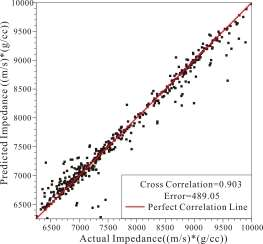Fig. 3. Cross plot of actual impedance and predicted impedance   Fig. 4. Cross Validation Result of Inversion. Correlation=0.832, Average Error=546.55[(m/s)*(g/cc)] Fig. 5. Arbitrary line from inversed impedance data volume. Base map is shown in the figure lowerleft. Table 1 Numerical analysis of inversion at well locations Training result Cross validation results correlation coefficient Average Error correlation coefficient Average Error 0.903 489.05 0.832 546.55 3.2 Seismic attributes selection by using multi-regression analysis Multi-regression analysis is a mathematical method which is used to analysis the relationship between one dependent variable and several independent variables (Hampson et al., 2001). The basic principle is that although there is no strict, deterministic functional relation between dependent variables and independent variables can try to find the most appropriate mathematical formula to express this relation. Multi-regression analysis can be used to solve the following problems: (1). Determine if there is correlation between certain variables. If it exists, find a suitable mathematical expression between them. (2). According to one or several variable values, predict the value of another variable, and calculate the forecast accuracy. (3). Factor analysis. For example, in the common effects of many variables for a variable, find out the most important factors, the secondary important factors, and the relationship between these factors. In the multi-regression analysis method, prediction error of N attributes is always less than or equal to N-1 attributes. Adding attributes means to use higher polynomial to fit curve. We can calculate the prediction error of each polynomial. This prediction error is equal to the root mean square error between real values and predicted values. With the increase of polynomial order, the prediction error decreases. But when we use overhigh order polynomial to fit curves, the existing data may fit well, but the interpolation or extrapolation over boundary would be fitting badly. This problem is called “over-trained”. In this study, the data would be divided into training data set and validation data set. The training data set is used to determine the correlation coefficient, and the validation data set is used to compute the validation error. If a high order polynomial fit the training data set well, but fit the validation data set badly. It means that the order of polynomial is too high. In this section, multi-regression analysis method is used to find the most suitable seismic attributes. As illustrated in Table 2, the training error gradually reduces with the increasing number of attributes, but when the number of attributes increases to four, validation error will rise. So, the best set of seismic attributes should contain three attributes that are the first three attributes in Table 2. The first three attributes are Inverted Impedance, Average Frequency and Filter 35/40-45/50. The most significant seismic attribute is Inverted impedance. Those attributes yield useful information about the lateral changes in lithology and porosity (Chopra and Marfurt, 2005). Furthermore, the training error for them is less than 3% that shows the exactness of results. It should be noted that PNN is a kind of nonlinear method, so the aforementioned attributes can be used as input for porosity prediction by PNN. (Kadkhodaie-Ilkhchi et al., 2009) Table 2 The result of multi-regression analysis for porosity estimation Final Attribute Training Error (%) Validation Error (%) Inverted Impedance 2.5677 2.6371 Average Frequency 2.4526 2.5881 Filter 35/40-45/50 2.4191 2.5672 Integrated Absolute Amplitude 2.3775 2.6071 Filter 25/30-35/40 2.3238 2.5925 Instantaneous Phase 2.2927 2.6179 Second Derivative Instantaneous Amplitude 2.2628 2.6973 3.3 Porosity estimation using PNN The main purpose of this section is to establish an optimum PNN model. The inputs of this model are three selected attributes in the previous section. In order to highlight the advantages of Probabilistic Neural Network in porosity estimation, another four algorithms have been used. Another four algorithms are single attribute analysis, multi-regression analysis, Multi-layer Feed Forward Network (MLFN) and Radial Basis Function (RBF). The training and validation results are shown in Table 3. According to the results, PNN algorithm gives less training and validation error. As seen from Table 3, the correlation coefficient of training result could reach 0.915, which is considered as a high correlation coefficient. It is higher than multi-regression analysis method (the correlation coefficient of multi-regression analysis is 0.844) and other methods. According to the numerical validation results, PNN method for porosity estimation is more accurate than others in this case. In the final of this section, the analysis for creating an optimum PNN model was done (Table 3 and Fig.6). Table 3 The training and validation results of neural networks method Training result Validation result correlation Error (%) correlation Error (%) single attribute analysis 0.822 2.568 0.811 2.637 multi-regression analysis 0.844 2.420 0.822 2.567 PNN 0.915 1.806 0.881 2.227 MLFN 0.864 2.280 0.848 2.676 RBF 0.886 2.086 0.772 2.963 Fig. 6. Cross plot of predicted porosity versus actual porosity 4. Results and Discussion We have demonstrated the application of Probabilistic Neural Networkto reservoir porosity estimation from seismic attributes. Two mathematical tools have been used: multi-regression analysis and PNN method. In the section of seismic impedance inversion, a qualified inverted impedance data volume has been calculated (Fig.3). In the section of seismic attributes selection, multi-regression analysis has been used to find appropriate seismic attributes (the first three attributes of Table 2). Those seismic attributes come from 3D seismic data volume and inverted impedance data volume. The optimal model is built up by PNN with proper trend and minimization of error. We have demonstrated this methodology on a set of 8 wells log data. The correlation coefficient of training data set could reach 0.915, which is considered as a high correlation coefficient (Fig.6). The well W5 is not used in training. It is used to validate the result of porosity estimation. The correlation coefficient of validation result could reach 0.881, which means that this methodology is reliable. The estimated porosity of W5 is displayed in Fig.7. After the establishment of an optimum PNN model for porosity estimation, we apply this model to all seismic data volume. Then, a porosity data volume could be calculated (Figs.8, 9). In Fig.9, an ancient river could be seen in the rectangle with higher porosity than elsewhere in the region. This is consistent with the law of geology. which shows, from one aspect, that the Probabilistic Neural Network is a reliable tool for porosity estimation. This method is an effective way to create an acceptable porosity data volume.   5. Conclusions We have demonstrated that the estimation of reservoir porosity from seismic attributes and inversion impedance using PNN method. In this study, two mathematic tools have been used: multi-regression analysis and PNN method. At attributes selection stage of this study, three attributes have been selected. At the porosity estimation stage, a PNN model has been established and trained. The training and validation correlation coefficient between predicted porosity and actual porosity could reach 0.915 and 0.881, respectively. The profile of estimated porosity shows that porosity variation in vertical direction is approximately increasing from bottom to the top and can be verified at well locations. The results indicate that PNN is a reliable method for porosity estimation. And it has obvious advantages in estimation accuracy compared with conventional methods such as multi-regression analysis and Multi-layer Feed Forward Network. The proposed methodology can be used to estimate porosity from seismic data. This methodology could reduce drilling risks and improve the success rate of exploration at the early stage of reservoir exploration. And it also could provide an acceptable porosity data volume which could be used to build reservoir geological model at the stage of reservoir development.

## Order a Unique Copy of this Paper

Essay Creek is an academic writing service provided to you by, a London-based company.

• Experience
Helping students successfully for 11 years.
• Confidentiality & Security
Be sure your information will be kept confidential due to our secure service.
• Quality & Reliability
8.5 out of 10 average quality score according to our customers' feedback. 97.45% of orders delivered on time.
• Versatility
478 active writers in 68 disciplines.
• 100% money back guarantee
You can always request a refund if you are not satisfied with the result.

Our team of writers is comprised of people with necessary academic writing skills and experience in various fields of study.

• Skilled writers only
We carefully choose writers to employ, paying attention to their skills and abilities.
• Competence
Your order will be assigned to a competent writer who specializes in your field of study.
• In-depth knowledge
Our writers know both peculiarities of academic writing and paper formatting rules.
• Motivation
We keep updated on results our writers show, motivating them to constantly improve their performance.